## 一 单层神经网络（感知器）

1.结构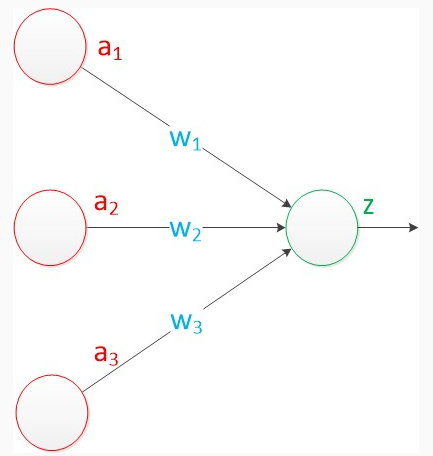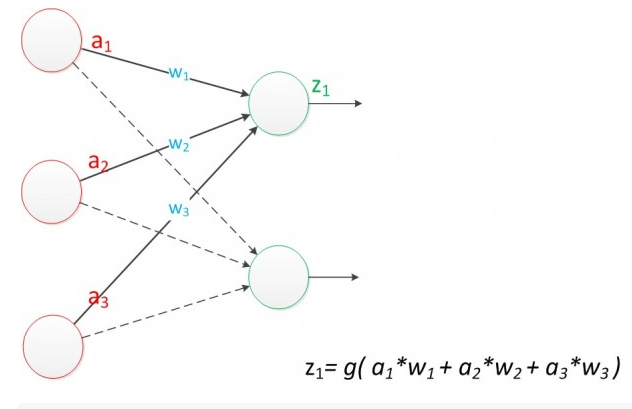图2 单层神经网络$$Z1$$图3 单层神经网络$$Z2$$图4 单层神经网络$$Z1和Z2$$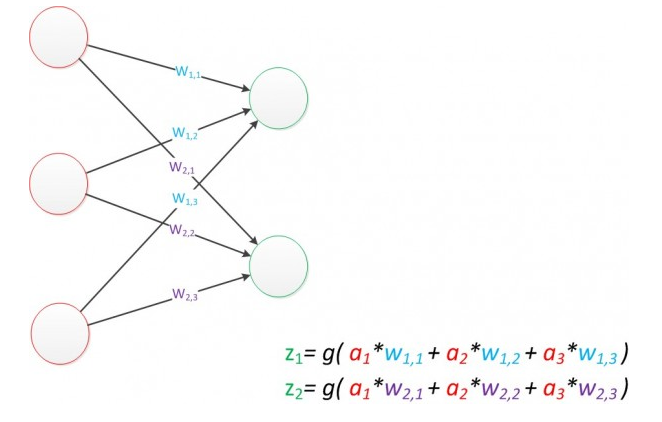图5 单层神经网络$$扩展$$


g(W * a) = z;

2.效果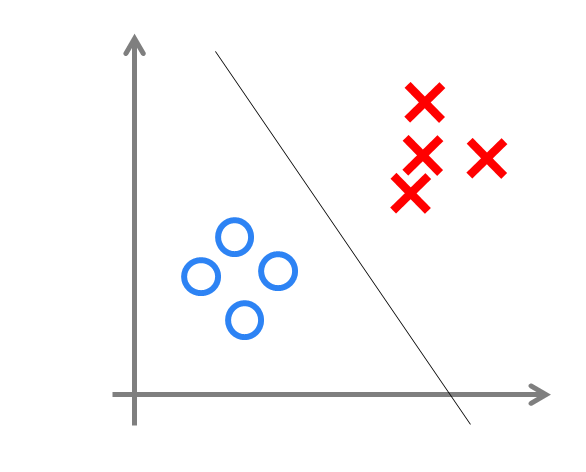图6 单层神经网络（决策分界）


## 二 两层神经网络（多层感知器）

1.结构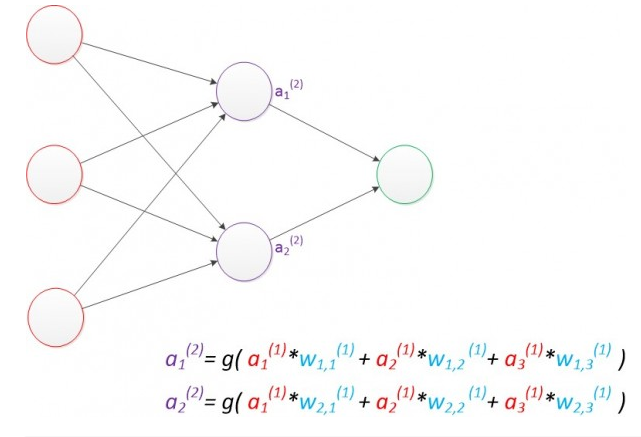图7 两层神经网络（中间层计算）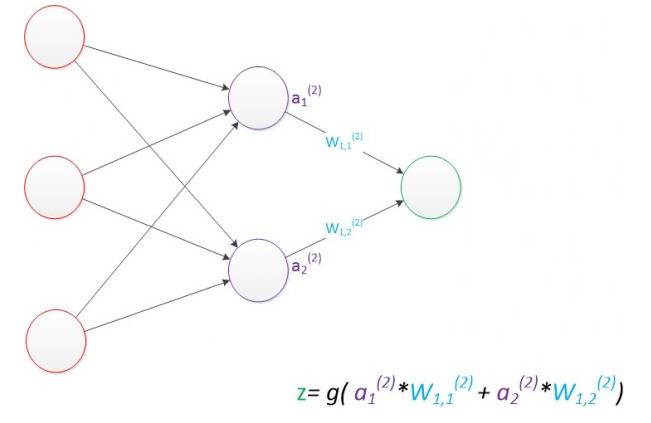图8 两层神经网络（输出层计算）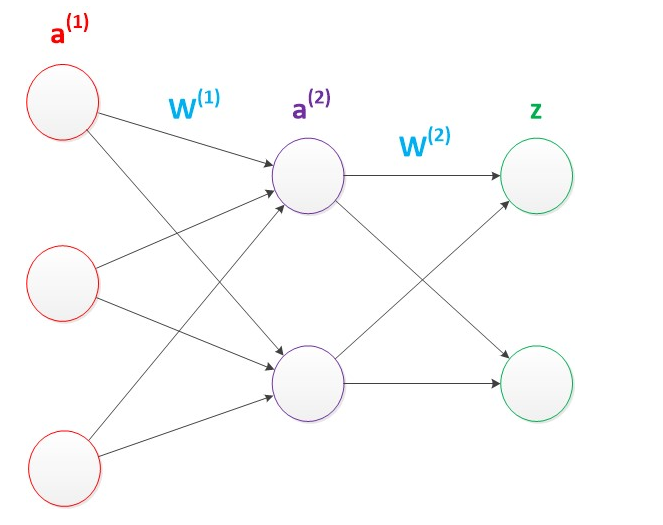图9 两层神经网络（向量形式）


g(W(1) * a(1)) = a(2);

g(W(2) * a(2)) = z;

                                   图10 两层神经网络（考虑偏置节点）


g(W(1) * a(1) + b(1)) = a(2);

g(W(2) * a(2) + b(2)) = z;

2.效果

                                      图11 两层神经网络（决策分界）


                                      图12 两层神经网络（空间变换）


                                      图13 EasyPR字符识别网络


EasyPR使用了字符的图像去进行字符文字的识别。输入是120维的向量。输出是要预测的文字类别，共有65类。根据实验，我们测试了一些隐藏层数目，发现当值为40时，整个网络在测试集上的效果较好，因此选择网络的最终结构就是120，40，65。

3.训练

loss = (yp - y)2

                                         图14 反向传播算法


## 三 多层神经网络（深度学习）

1.结构

                                      图15 多层神经网络



g(W(1) * a(1)) = a(2);

g(W(2) * a(2)) = a(3);

g(W(3) * a(3)) = z;

多层神经网络中，输出也是按照一层一层的方式来计算。从最外面的层开始，算出所有单元的值以后，再继续计算更深一层。只有当前层所有单元的值都计算完毕以后，才会算下一层。有点像计算向前不断推进的感觉。所以这个过程叫做“正向传播”。


                                   图16 多层神经网络（更深的层次）


2.效果

                                   图17 多层神经网络（特征学习）


3.训练